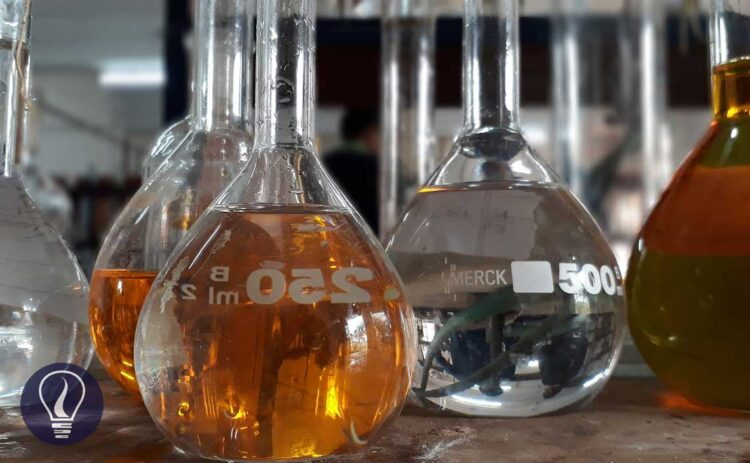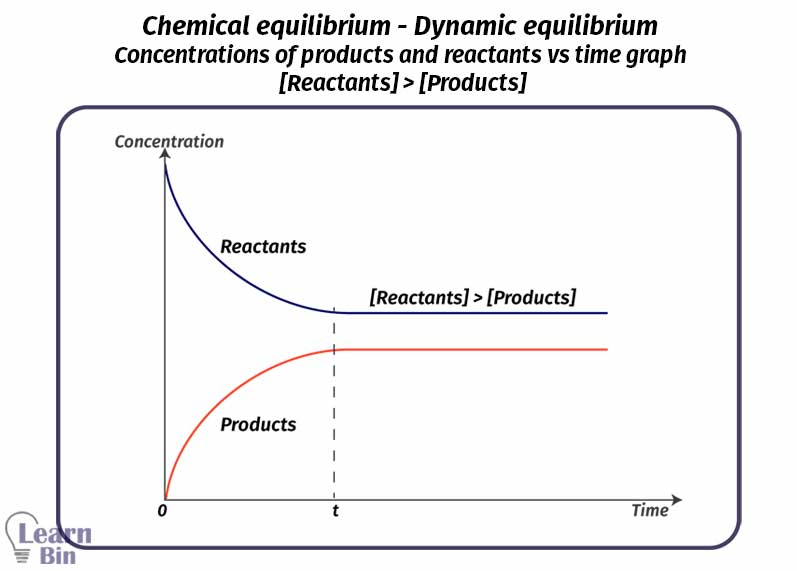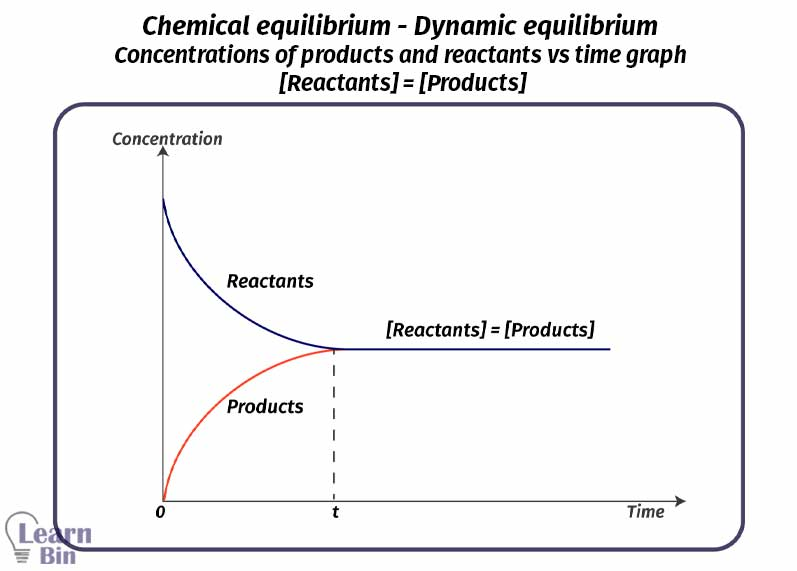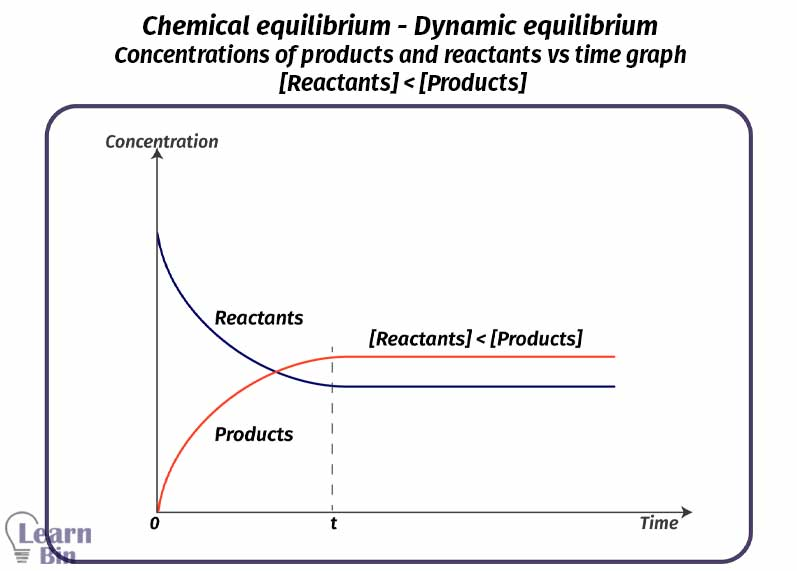More results...

Generic selectors
Exact matches only
Search in title
Search in content
Post Type Selectors

# What is an Equilibrium System - Introduction to Chemical EquilibriumB.Sc (Hons, USJ) (Polymer Science and Technology, Chemistry, Physics)
Categories
Last Updated On : January 20, 2023
Published Date : October 10, 2022

## What is an Equilibrium System?

When the rate of formation of a component in a system is equal to the rate of consumption of that component, the system is said to be in a steady state. Here, macroscopic properties are not changed with time. The properties measured considering the system as a whole, are called macroscopic properties. Let’s consider a few steady systems,

• Considering a uniformly burning candle, the reason why the properties of the flame remain unchanged is that the rates of the materials entering and leaving it are the same.
• When the water in a water tank is drained at the same rate and filled with water at the same rate, there is no change in the total volume.

But the above systems are not equilibrium systems. To be an equilibrium system, there should be,

1. There should be a reversible reaction. That means the conversion of reactants into products and products into reactants happens in the same system.
2. The system should be a closed system. There is no exchange of matter through the system boundary.
3. The temperature of the system should be constant over time.

Let’s consider the following reaction,

A ↔ B

In the beginning, component A is added to a closed system. An amount of mols from component A is converted into product B. the rate of formation of B is maximum in the beginning. The rate is being decreased with time. In the beginning, there is no B in the system. But after B is formed, some mols of B are converted to component A,

Because there is no B in the beginning, the rate of formation of A from B is zero. But that rate increased with time. After a while when the temperature is constant, the rate of "forward reaction" and the rate of reverse reaction will be equal. Both reactions occur at the same rate if there is no external influence. At this point, the system is said to be in ' dynamic equilibrium '.

Rf = Rr

• Rf = rate of the forward reaction
• Rr = rate of the reverse reaction

In an equilibrium system, there are both reactants and products. In this system, macroscopic properties such as mols, mol fractions, and concentration remain constant. After became equilibrium there are three types of relationships between the concentrations of products and reactants.

[Reactants] > [Products]

[Reactants] = [Products]

[Reactants] < [Products]Figure 02: Chemical equilibrium - Dynamic equilibrium - Concentrations of products and reactants vs time graph - [Reactants] > [Products]Figure 03: Chemical equilibrium - Dynamic equilibrium - Concentrations of products and reactants vs time graph - [Reactants] = [Products]Figure 04: Chemical equilibrium - Dynamic equilibrium - Concentrations of products and reactants vs time graph - [Reactants] < [Products]

## le Chatelier's principle

If an external influence occurs in a balanced system in such a way that the equilibrium is broken, the system will return to equilibrium by carrying out more of the forward or backward reaction and equalizing the reaction rates. External influences are like concentration of each component, temperature, partial pressure, etc.

The equilibrium point for any reaction is not fixed. It changes when the reaction conditions are changed. In a system in dynamic equilibrium, if any factor that brought about that equilibrium is inhibited, the system will rearrange itself so that the inhibition is minimized.## References and Attributes

### Figures:

The cover image was created using an image by Tuhin khamaru from Pixabay